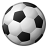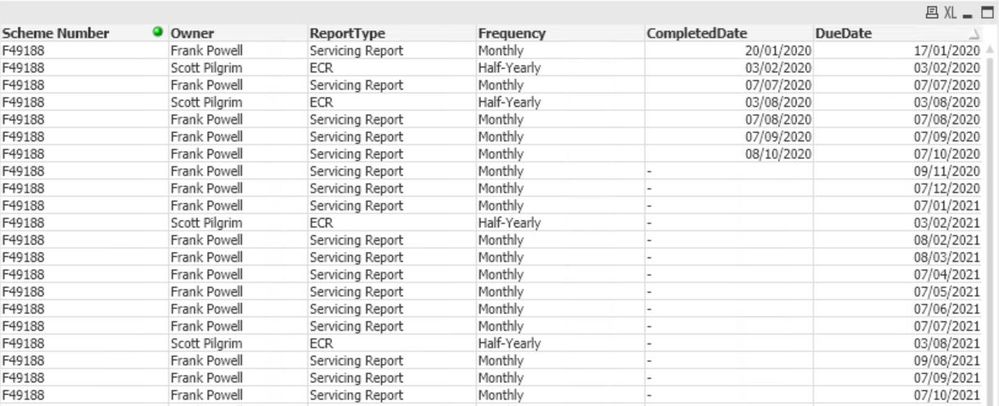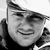# QlikView App Dev

Discussion Board for collaboration related to QlikView App Development.

cancel
Showing results for
Did you mean:Creator III

Hello everyone,

I'm having difficulty trying to transform a table of data I have, the script is a simple straight load which gives me data like this;This is how the flat table looks

I need to transform this so I end up with a unique line for each entry that shows the max completed date and then 2 more fields with next due, next due +1. So it should look like the picture below;Transformed Table

If anyone can help me out I'd be massively thankful - I'm not a million miles away, I just can't quite get it nailed.

Labels (1)
• ### QLIKVIEW help

2 Solutions

Accepted SolutionsMVP

You need to aggregate in some way. Try to create a table using all the dimensions except the two date dimensions. Then for maxCompletedDueDate use this expression

=MaxString(CompletedDate)

For the due dates try the max function

=Min(DueDate)

=min(DueDate, 2)MVP

To exclude the completed you also might need to adjust your expression with a SET modifier.

=Min({<CompletedDate - ={*} >} DueDate)

=min({<CompletedDate - ={*} >}DueDate, 2)

2 RepliesMVP

You need to aggregate in some way. Try to create a table using all the dimensions except the two date dimensions. Then for maxCompletedDueDate use this expression

=MaxString(CompletedDate)

For the due dates try the max function

=Min(DueDate)

=min(DueDate, 2)MVP

To exclude the completed you also might need to adjust your expression with a SET modifier.

=Min({<CompletedDate - ={*} >} DueDate)

=min({<CompletedDate - ={*} >}DueDate, 2)